# NS-CUK Joint Journal Club : S.T.Nguyen, Review on "Graph Neural Networks for Link Prediction with Subgraph Sketching", ICLR 2023.

26. May 2023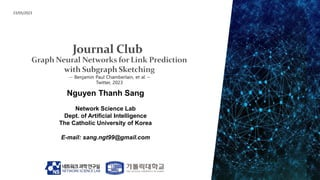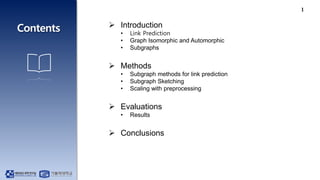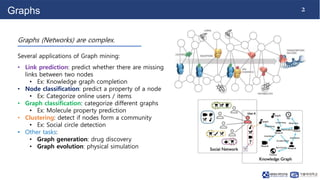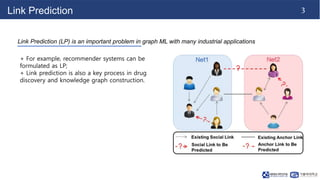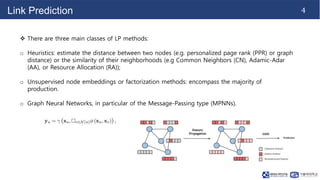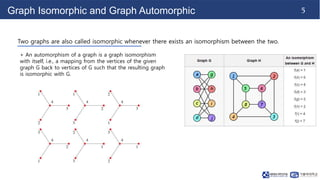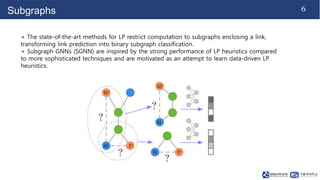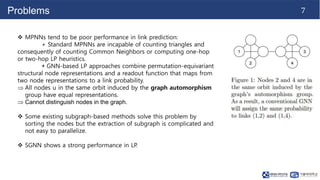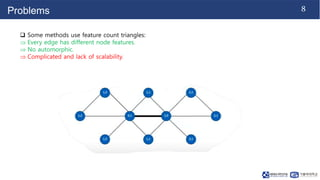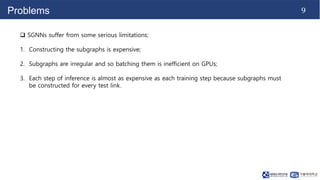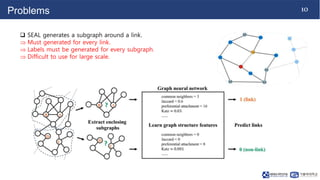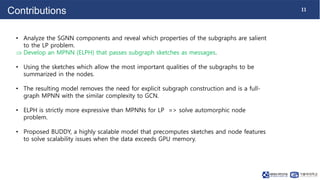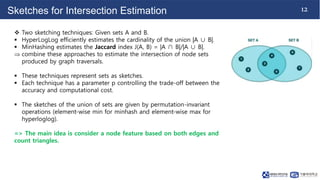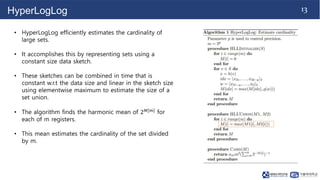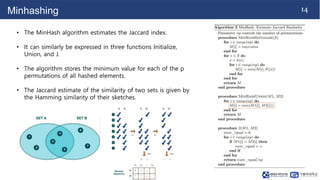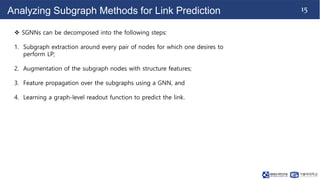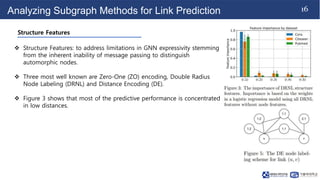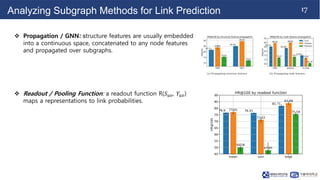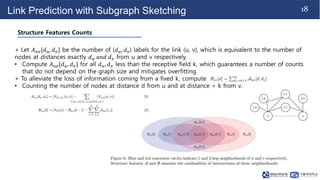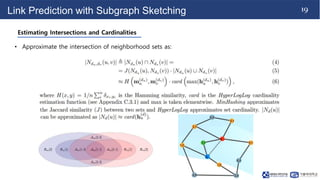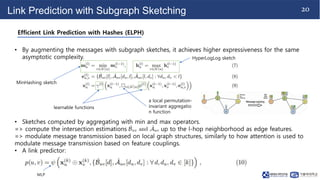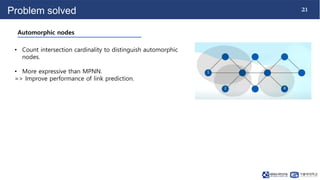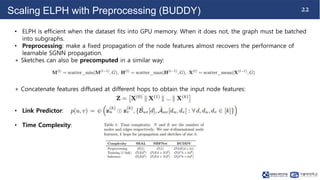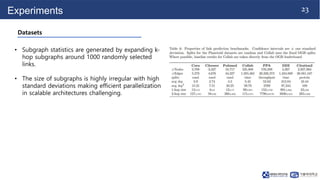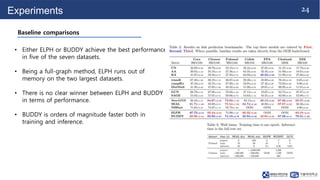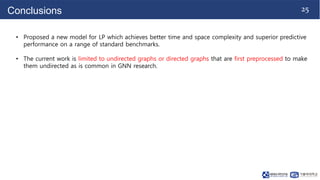1 von 27

### NS-CUK Joint Journal Club : S.T.Nguyen, Review on "Graph Neural Networks for Link Prediction with Subgraph Sketching", ICLR 2023.

• 1. Nguyen Thanh Sang Network Science Lab Dept. of Artificial Intelligence The Catholic University of Korea E-mail: sang.ngt99@gmail.com 23/05/2023
• 2. 1  Introduction • Link Prediction • Graph Isomorphic and Automorphic • Subgraphs  Methods • Subgraph methods for link prediction • Subgraph Sketching • Scaling with preprocessing  Evaluations • Results  Conclusions
• 3. 2 Graphs Graphs (Networks) are complex. Several applications of Graph mining: • Link prediction: predict whether there are missing links between two nodes • Ex: Knowledge graph completion • Node classification: predict a property of a node • Ex: Categorize online users / items • Graph classification: categorize different graphs • Ex: Molecule property prediction • Clustering: detect if nodes form a community • Ex: Social circle detection • Other tasks: • Graph generation: drug discovery • Graph evolution: physical simulation
• 4. 3 Link Prediction Link Prediction (LP) is an important problem in graph ML with many industrial applications + For example, recommender systems can be formulated as LP; + Link prediction is also a key process in drug discovery and knowledge graph construction.
• 5. 4 Link Prediction  There are three main classes of LP methods: o Heuristics: estimate the distance between two nodes (e.g. personalized page rank (PPR) or graph distance) or the similarity of their neighborhoods (e.g Common Neighbors (CN), Adamic-Adar (AA), or Resource Allocation (RA)); o Unsupervised node embeddings or factorization methods: encompass the majority of production. o Graph Neural Networks, in particular of the Message-Passing type (MPNNs).
• 6. 5 Graph Isomorphic and Graph Automorphic Two graphs are also called isomorphic whenever there exists an isomorphism between the two. + An automorphism of a graph is a graph isomorphism with itself, i.e., a mapping from the vertices of the given graph G back to vertices of G such that the resulting graph is isomorphic with G.
• 7. 6 Subgraphs + The state-of-the-art methods for LP restrict computation to subgraphs enclosing a link, transforming link prediction into binary subgraph classification. + Subgraph GNNs (SGNN) are inspired by the strong performance of LP heuristics compared to more sophisticated techniques and are motivated as an attempt to learn data-driven LP heuristics.
• 8. 7 Problems  MPNNs tend to be poor performance in link prediction: + Standard MPNNs are incapable of counting triangles and consequently of counting Common Neighbors or computing one-hop or two-hop LP heuristics. + GNN-based LP approaches combine permutation-equivariant structural node representations and a readout function that maps from two node representations to a link probability.  All nodes u in the same orbit induced by the graph automorphism group have equal representations.  Cannot distinguish nodes in the graph.  Some existing subgraph-based methods solve this problem by sorting the nodes but the extraction of subgraph is complicated and not easy to parallelize.  SGNN shows a strong performance in LP.
• 9. 8 Problems  Some methods use feature count triangles:  Every edge has different node features.  No automorphic.  Complicated and lack of scalability.
• 10. 9 Problems  SGNNs suffer from some serious limitations: 1. Constructing the subgraphs is expensive; 2. Subgraphs are irregular and so batching them is inefficient on GPUs; 3. Each step of inference is almost as expensive as each training step because subgraphs must be constructed for every test link.
• 11. 10 Problems  SEAL generates a subgraph around a link.  Must generated for every link.  Labels must be generated for every subgraph.  Difficult to use for large scale.
• 12. 11 Contributions • Analyze the SGNN components and reveal which properties of the subgraphs are salient to the LP problem.  Develop an MPNN (ELPH) that passes subgraph sketches as messages. • Using the sketches which allow the most important qualities of the subgraphs to be summarized in the nodes. • The resulting model removes the need for explicit subgraph construction and is a full- graph MPNN with the similar complexity to GCN. • ELPH is strictly more expressive than MPNNs for LP => solve automorphic node problem. • Proposed BUDDY, a highly scalable model that precomputes sketches and node features to solve scalability issues when the data exceeds GPU memory.
• 13. 12 Sketches for Intersection Estimation  Two sketching techniques: Given sets A and B.  HyperLogLog efficiently estimates the cardinality of the union |A ∪ B|.  MinHashing estimates the Jaccard index J(A, B) = |A ∩ B|/|A ∪ B|.  combine these approaches to estimate the intersection of node sets produced by graph traversals.  These techniques represent sets as sketches.  Each technique has a parameter p controlling the trade-off between the accuracy and computational cost.  The sketches of the union of sets are given by permutation-invariant operations (element-wise min for minhash and element-wise max for hyperloglog). => The main idea is consider a node feature based on both edges and count triangles.
• 14. 13 HyperLogLog • HyperLogLog efficiently estimates the cardinality of large sets. • It accomplishes this by representing sets using a constant size data sketch. • These sketches can be combined in time that is constant w.r.t the data size and linear in the sketch size using elementwise maximum to estimate the size of a set union. • The algorithm finds the harmonic mean of 2𝑀[𝑚] for each of m registers. • This mean estimates the cardinality of the set divided by m.
• 15. 14 Minhashing • The MinHash algorithm estimates the Jaccard index. • It can similarly be expressed in three functions Initialize, Union, and J. • The algorithm stores the minimum value for each of the p permutations of all hashed elements. • The Jaccard estimate of the similarity of two sets is given by the Hamming similarity of their sketches.
• 16. 15 Analyzing Subgraph Methods for Link Prediction  SGNNs can be decomposed into the following steps: 1. Subgraph extraction around every pair of nodes for which one desires to perform LP; 2. Augmentation of the subgraph nodes with structure features; 3. Feature propagation over the subgraphs using a GNN, and 4. Learning a graph-level readout function to predict the link.
• 17. 16 Analyzing Subgraph Methods for Link Prediction  Structure Features: to address limitations in GNN expressivity stemming from the inherent inability of message passing to distinguish automorphic nodes.  Three most well known are Zero-One (ZO) encoding, Double Radius Node Labeling (DRNL) and Distance Encoding (DE).  Figure 3 shows that most of the predictive performance is concentrated in low distances. Structure Features
• 18. 17 Analyzing Subgraph Methods for Link Prediction  Propagation / GNN: structure features are usually embedded into a continuous space, concatenated to any node features and propagated over subgraphs.  Readout / Pooling Function: a readout function R(𝑆𝑢𝑣, 𝑌𝑢𝑣) maps a representations to link probabilities.
• 19. 18 Link Prediction with Subgraph Sketching + Let 𝐴𝑢𝑣[𝑑𝑢, 𝑑𝑣] be the number of (𝑑𝑢, 𝑑𝑣) labels for the link (u, v), which is equivalent to the number of nodes at distances exactly 𝑑𝑢 𝑎𝑛𝑑 𝑑𝑣 from u and v respectively. • Compute 𝐴𝑢𝑣[𝑑𝑢, 𝑑𝑣] for all 𝑑𝑢, 𝑑𝑣 less than the receptive field k, which guarantees a number of counts that do not depend on the graph size and mitigates overfitting. + To alleviate the loss of information coming from a fixed k, compute • Counting the number of nodes at distance d from u and at distance > k from v. Structure Features Counts
• 20. 19 Link Prediction with Subgraph Sketching • Approximate the intersection of neighborhood sets as: Estimating Intersections and Cardinalities
• 21. 20 Link Prediction with Subgraph Sketching • By augmenting the messages with subgraph sketches, it achieves higher expressiveness for the same asymptotic complexity. • Sketches computed by aggregating with min and max operators. => compute the intersection estimations up to the l-hop neighborhood as edge features. => modulate message transmission based on local graph structures, similarly to how attention is used to modulate message transmission based on feature couplings. • A link predictor: Efficient Link Prediction with Hashes (ELPH) learnable functions MinHashing sketch HyperLogLog sketch a local permutation- invariant aggregatio n function MLP
• 22. 21 Problem solved • Count intersection cardinality to distinguish automorphic nodes. • More expressive than MPNN. => Improve performance of link prediction. Automorphic nodes
• 23. 22 Scaling ELPH with Preprocessing (BUDDY) • ELPH is efficient when the dataset fits into GPU memory. When it does not, the graph must be batched into subgraphs. • Preprocessing: make a fixed propagation of the node features almost recovers the performance of learnable SGNN propagation. + Sketches can also be precomputed in a similar way: + Concatenate features diffused at different hops to obtain the input node features: • Link Predictor: • Time Complexity:
• 24. 23 Experiments • Subgraph statistics are generated by expanding k- hop subgraphs around 1000 randomly selected links. • The size of subgraphs is highly irregular with high standard deviations making efficient parallelization in scalable architectures challenging. Datasets
• 25. 24 Experiments • Either ELPH or BUDDY achieve the best performance in five of the seven datasets. • Being a full-graph method, ELPH runs out of memory on the two largest datasets. • There is no clear winner between ELPH and BUDDY in terms of performance. • BUDDY is orders of magnitude faster both in training and inference. Baseline comparisons
• 26. 25 Conclusions • Proposed a new model for LP which achieves better time and space complexity and superior predictive performance on a range of standard benchmarks. • The current work is limited to undirected graphs or directed graphs that are first preprocessed to make them undirected as is common in GNN research.
• 27. 26